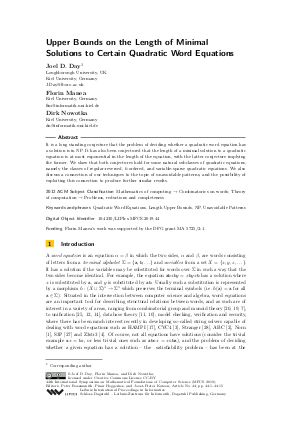Document# Upper Bounds on the Length of Minimal Solutions to Certain Quadratic Word Equations

### Authors Joel D. Day, Florin Manea, Dirk Nowotka## File

LIPIcs.MFCS.2019.44.pdf
• Filesize: 0.5 MB
• 15 pages

## Cite As

Joel D. Day, Florin Manea, and Dirk Nowotka. Upper Bounds on the Length of Minimal Solutions to Certain Quadratic Word Equations. In 44th International Symposium on Mathematical Foundations of Computer Science (MFCS 2019). Leibniz International Proceedings in Informatics (LIPIcs), Volume 138, pp. 44:1-44:15, Schloss Dagstuhl - Leibniz-Zentrum für Informatik (2019)
https://doi.org/10.4230/LIPIcs.MFCS.2019.44

## Abstract

It is a long standing conjecture that the problem of deciding whether a quadratic word equation has a solution is in NP. It has also been conjectured that the length of a minimal solution to a quadratic equation is at most exponential in the length of the equation, with the latter conjecture implying the former. We show that both conjectures hold for some natural subclasses of quadratic equations, namely the classes of regular-reversed, k-ordered, and variable-sparse quadratic equations. We also discuss a connection of our techniques to the topic of unavoidable patterns, and the possibility of exploiting this connection to produce further similar results.

## Subject Classification

##### ACM Subject Classification
• Mathematics of computing → Combinatorics on words
• Theory of computation → Problems, reductions and completeness
##### Keywords
• Length Upper Bounds
• NP
• Unavoidable Patterns

## Metrics

• Access Statistics
• Total Accesses (updated on a weekly basis)
0